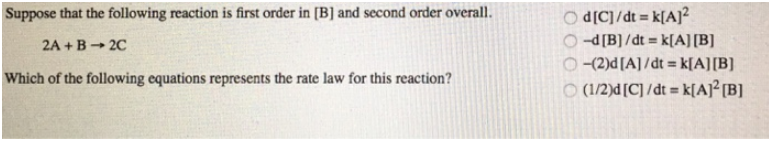# Problem: Suppose that the following reaction is first order in [B] and second order overall. 2A + B → 2C Which of the following equations represents the rate law for this reaction? (a) d[C]/dt = k[A]2 (b) -d[B]/dt = k[A][B] (c) -(2)d[A]/dt = k[A][B] (d) (1/2)d[C]/dt = k[A]2[B]

###### FREE Expert Solution
92% (372 ratings)###### Problem Details

Suppose that the following reaction is first order in [B] and second order overall.

2A + B → 2C

Which of the following equations represents the rate law for this reaction?

(a) d[C]/dt = k[A]2

(b) -d[B]/dt = k[A][B]

(c) -(2)d[A]/dt = k[A][B]

(d) (1/2)d[C]/dt = k[A]2[B]